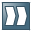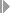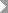Show TOC

###Calculating Variances#### Procedure

1. ChooseAccountingControllingProduct Cost ControllingCost Object ControllingProduct Cost by Period or Product Cost by OrderPeriod-End Closingor . Then choose the following:

• In Product Cost by Period choose Single Functions: Product Cost Collector orSingle Functions: Cost Object HierarchyVariancesIndividual Processing or Collective Processing.

• In Product Cost by Order, chooseVariancesIndividual Processing or Collective Processing.

To calculate the variances for a particular order (product cost collector or manufacturing order) choose Individual Processing. To calculate the variances for all product cost collectors in a plant, choose Collective Processing.

To calculate the variances for a single cost object hierarchy, choose Individual Processing. To calculate the variances for all cost object hierarchies in a controlling area, choose Collective Processing.

2. Enter data as required.

3. Specify whether you want to calculate the variances for all target cost versions that you defined in Customizing, or only for a particular target cost version. If you choose Selected target cost versions, specify the target cost version underExtrasSet versions.

4. Set the indicators for processing control as desired.Note

Only the variances calculated on the basis of target cost version 0 can be settled to Profitability Analysis (CO-PC).

End of the note.
5. ChooseVariance CalculationExecute.

#### Result

The system calculates the variances. If you have set the Detail list indicator in the processing options, the screen Variance Calculation: List appears.

This is a list of all processed objects and their values. It includes:

• The target costs (according to the target cost version)

• The posted actual costs (debits for goods issues, internal activity allocations, overhead, and so forth)

• The allocated actual costs (credits due to goods receipts and settlements)

• The sum of the variances on the input sides

• The sum of the variances

You can use the display variant to display other fields (such as the scrap variance).Note

For information on when you should select the Detail list indicator, refer to the section Variance Calculation under Features. If you didn't select the indicator, the Basic List screen appears.

The information shown in the basic list includes:

• The selection parameters

• The total number of messages and the number of messages of each message type

• The processing categories

End of the note.

If the variances were calculated in a target cost version relevant to settlement, the order receives the status VCAL (Variances calculated). You can include this status in the definition of the status profile in Customizing for the Product Cost Controlling Information System.Note

If an order (product cost collector or manufacturing order) is not processed in collective processing, access individual processing and start variance calculation for the individual product cost collector. The system issues messages in individual processing that are suppressed in collective processing.

End of the note.
##### Breakdown of Variances into Variance Categories

To see the variances broken down into variance categories, position the cursor on the value in the variances column and double-click.

##### Breakdown of Variances into Cost Elements

To see the variances broken down into cost elements, chooseGo toCost Elementsfrom the object list. The screen Variance Calculation: List appears again, but this time the costs are broken down into cost elements. If you have selected the Material origin indicator in the costing view of the master records of the materials, you can continue to expand the cost elements for materials and see the variance at the material level.

##### Explanation of the Target Costs

To see how the target costs were calculated, position the cursor on a value in the target costs column and chooseExtrasExplain target costs. The screen Target Cost Calculation: Explanation appears.

##### Explanation of the Variances

To see how the variances were calculated by cost element, position the cursor on a value in the column variances in the cost element display, and chooseExtrasExplain variances. The screen Variance Calculation: Explanation appears.

Clicking on a value for the variance category in the Total column gives you information on how the value for that variance category and cost element was calculated.

##### Explanation of the Scrap Variance

To see how the scrap variance was calculated for each cost element, chooseExtrasScrap analysis. From the screen Variance Calculation: List you can access the explanation facility for scrap. Here you can see which operations incurred scrap variances, and the costs.

##### Switching to a Different Target Cost Version

If you have calculated the costs for multiple target cost versions and want to switch to a different target cost version, chooseGo toTarget Cost Versionand choose the desired target cost version.

##### Basic List

When you calculate the variances for a cost object hierarchy, you first come to the screen Variance Calculation Cost Object Hierarchy: Basic List.

##### Hierarchy List

To view the cost object hierarchy, chooseGotoHierarchy List.

This displays all cost object nodes in the cost object hierarchy.

The functions Expand Nodes and Collapse Nodes are available for navigating through the cost object hierarchy.

##### Cost Element Breakdown

Double-click on a hierarchy node to bring up the screen Variance Calculation for Cost Object Hierarchy: List. The header contains information on the controlling area, processing status (update run, test run) and so forth.

The following data for the input side and the output side is displayed for each cost element on each object below the selected cost object node:

• Target costs

• Actual costs

• Confirmed quantity

• Total variance

The field Processing step indicates what data the system is displaying:

• Original data

The original data is the actual costs that were posted to the cost object node and the target costs that were calculated on the basis of the standard cost estimates for the materials.

• Processed data

The processed data is the actual costs and the target costs that were summarized during variance calculation or distributed before the variances were calculated. In this case, the actual costs are the total costs that were posted on the cost object node and summarized on the higher hierarchy levels or distributed to the lower-level orders.

##### Totals List

From the hierarchy list, you can display the cumulative costs for each node of the cost object hierarchy by selecting the node and choosingGotoTotals list.

The screen Variance Calculation Cost Object Hierarchy: List appears. The list shows the following information for each node of the hierarchy:

• Target costs (according to the target cost version)

• Actual costs (charges to the cost object)

• Allocated actual costs (credits for goods receipts and settlements)

• Variances

For each line in the list, you can useGotoDetailto access detailed information on the selected node, such as the posted actual costs, scrap, work in process, allocated actual costs, and information on the variance categories. This information is structured according to fixed costs, variable costs, and total costs.Note

In order for the fixed costs to be separated from the variable costs, the price control indicator for the material must be set to S (standard price) and the material must have a current standard cost estimate.

End of the note.
##### Schedule Manager: Worklist Monitor

If you are using the multilevel worklist, you can edit the objects that have errors in the monitor of the worklist. For more information, see the following section: